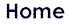Philosophy of Science Philosophy of Contents: Form and Coulomb's Law Leonid G. Kreidik Polytechnic Academy, Minsk, Belarus George P. ShpenkovTechnical University, Bydgoszcz, Poland gshp@atv.bydgoszcz.plABSTRACT: In this paper I conduct a philosophical-physical analysis leading to the development of a philosophically justified form of Coulomb's law on the basis of contents-form philosophy.. From this it follows that dimensionality of "electric charge" at the subatomic level of matter is g/s, i.e., the charge in fact represents the mass speed of exchange at the field level. Thus, the philosophic-logical solution to Coulomb's law on the basis of contents-form philosophy radically changes our conventional concepts about the microworld, the consequences of which will be considered in greater detail.I. Introduction The purpose of this article is to demonstrate how disregarding the philosophical approach at solving physics problems, on the basis of a concrete example of dimensionality establishment, leads to serious consequences, and to what extent. A transformation of the dimensionalities of physical quantities, for both electric as well as magnetic fields with fractional powers of CGS units (that are the basis for SI units) into dimensionalities with integer powers of the units, gets at present exclusively important meaning, especially, after the disaster happened at Chernobyl's atomic station. An understanding of nature of the electric charge and electromagnetic field, more profound penetration in the essence of atom structure depends on solving this problem that has to promote in the nearest future an atomic technologies perfecting. Numerous attempts in the first half of 20th century to solve the dimensionality problem by physics itself have met with failure. The reason is that the problem lies on the boundary of philosophy and physics and can be solved only on the basis of the philosophic-physical analysis. We examine this problem using our solutions . II. Philosophy of Basic Concepts of Measures A. As is well known, physical measurement of a quantity A is the comparison expressed by the relation Ar = A / EA. Here, A is an absolute composite multiplicative measure equal to the product of components A1, A2, ..., An (which are not necessarily have to be elementary) of this measure, A = A1 · A2 ··· An; Ar = A1r · A2r ···Anr is an relative composite measure; EA = EA1 · EA2 ··· EAn is a composite unit of measurement. Thus, the absolute measure M(A) of the physical quantity A is equal to the product M(A) = Ar · EA or briefly A = Ar · EA.(1) Any constituent measure represents some direct Y or inverse 1/Y measure which can be both absolute and relative. In physics, the composite quantities A and EA are termed the derivative quantities. Basic elementary absolute measures are the measure of contents represented by mass, the measure of form expressed by length, and the measure of time defined by duration. We adopt as base or reference units of the elementary absolute measures the unit of mass the gram (g), the unit of length the centimeter (cm), and the unit of time the second (s). C. In a general case, the physical symbols A and EA have three logical meanings: 1) a name Z or a nomination (from the Latin, nomination=name), we denote it conditionally by equalities nomA = Z and nomEA = EZ (it is intended to be read "a nomination of the unit EA of the physical quantity A is EZ"); 2) a measure A and EA; 3) a dimensionality or dimensio (from the Latin, dimensio=measurement), dimA and dimEA, which determines the structure of the measures A and EA on the basis of the reference units,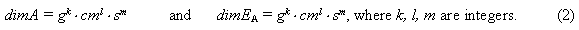Thus, in a general case, a physical symbol F represents a trisignification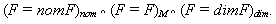Here, subscripts nom, M and dim indicate, respectively, the name, measure and dimensionality. A number of trisignifications of the same quantity is defined by the number of theories which can describe this quantity. The symbol F of a physical quantity is the symbolic form of representation of its name and measure, but a dimensio constitutes its contents. The last have to be supplemented by a qualitative (textual) contents-description that is usually initial. The dimensio itself as a physical symbol is, on the one hand, the nomination gk · cml · sm of the composite unit and, on the other hand, is the composite structural unit 1 gk · cml · sm. If EA is a reference unit then its nomination and dimensionality coincide,In a case of a composite unit, its nomination and dimensionality do not coincide nominatively, but as the units they are equal,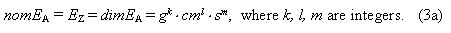Strictly speaking, the expression (3a) should be represented in the form of the following disignificationThe above disignification states: as to dimensionalities, the expressions EZ and gk · cml · sm are equal since they represent the same unit, but as to nominations, these expresions are not equal because they are expressed by different names. On the basis of nominations Z1, Z2, ..., Zn, as composite units of measurement, the nominative dimensionality of the composite unit EA,is formed. If make use the metric units, the formula of dimensionality for the absolute-relative derivative unit EA takes the following form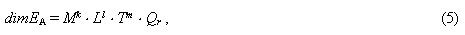where the main metric units of mass M, length L, and time T are decimal-multiple to the base units or compose their decimal parts; k, l, m are integers; Qr is the relative component of the derivative unit, which in a general case is a product of s-cofactors of relative units with integer, rational and irrational powers: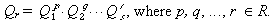If in the formula (5) k = l = m = 0 to be assumed, the derivative unit EA becomes a relative one and in this sense the relative unit is the absolute zero-dimensional unit or, simply, the unit of zero dimensionality. We term dimensionality of the kind (5) the rational dimensionality because the reference units are contained in it in the form of integer powers. A system of units built on the basis of rational dimensionalities of reference units has termed the GCS system of units . Derivative units contained at least one fractional power of a reference unit, we term irrational units because they contain the reference units under the root sign: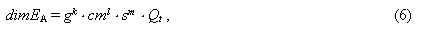where k, l, m are rational numbers and Qt is a quantitative component of the unit EA. A system of units admitting the irrational dimensionalities on the basis of the reference units g, cm, and s is termed CGS system of units. The CGS-system is the base of modern units (SI units) describing, in particular, electric and magnetic phenomena. C. We term the rational and irrational units, correspondingly, theoretical and phenomenological units. The phenomenological units have not been obtained on the basis of comparison, they were generated in physics of electric and magnetic phenomena by Coulomb's law. Fractional powers of reference units, being in the phenomenological measures, exclude a possibility of the philosophic and physical understanding of being investigated object or phenomenon. Indeed, at present, nobody in science can show an object with dimensionality, etc. In particular, the phenomenological unit of electrical charge with dimensionalityexcludes understanding its nature. Irrational dimensionalities can formally hide by means of nominative dimensionalities, but it is impossibly to eliminate them from the theories given rise to them. D. Physical quantities of the same absolute dimensionality of the GCS-system express the different manifestation of the same property of a multiface process or an object of study. We refer their, according to Danchev , to the same class of physical quantities designated by the symbol D(k, l, m), where k, l, m are arguments of the class - integers, contained in the formula of dimensionality (5). The first number k is the argument of contents, the second number l is the argument of form and the third number m is the argument of time. We will term the definite classes of physical quantities briefly the Danchev's classes or, simply, classes. All absolute physical quantities belonging to the different classes are different, although incorrect states of an opposite character sometimes occurr. E. Physical quantities belonging to the classes with the unit argument of contents D(1, l, m) form a set of dynamic physical quantities or, simply, the dynamic set. Physical quantities with the zero argument of contents and different arguments of form and time, D(0, l, m), form a kinematic set of physical quantities or, briefly, the kinematic set. We introduce the absolute unit density of contents (substance) e0 by the expression e0=1 g/cm3, then an arbitrary density of contents will be presented by the product e = e0er, where er is the relative density of contents. The quantity m, inverse to the density of contents, we term the density of form m = 1/e = m0mr, where m0=1/e0=1 cm3/g is the absolute unit density of form (volume) and mr = 1/er is the relative density of form. Composite physical quantities A and B of dynamic and kinematic sets ,correspondingly, which are related by the equality A=e0e rB or B=m0mrA, we term the conjugate in contents-form (form-contents) quantities. They can also be expressed by the relation A=e0Av, where Av=erB is a kinematic quantity. F. Because of the fully developed historical error related to the confusion with the notions of electric current I and its circulation G = (1/c) I , the conjugated in speed of light c pairs of quantities a and A (I and G) of the same nomination but the different nature had appeared. They can be expressed by the disignificationwhereand c = crev is the speed of light expressed by the unit speed ev=1 cm/s and by the relative speed cr = c/ev = 2.99792458 · 1010. The notion circulation G characterizes the magnetic field strength of current, its theory is presented in the work . The quantities a and A, having relation to the electric and magnetic fields, have got the terms of electric and magnetic quantities, correspondingly. The conjugated pairs a and A are usually designated by the same symbol a or A that generates the disignification of nomination (A = a)E ^ (A = a)B; here, subscripts E and B point to the electric and magnetic quantities, respectively. Consequently, in electromagnetic theory, the physical symbol A is described by two trisignifications, which have generated by the disignification of nomination: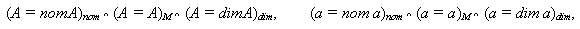The both trisignifications represent hexasignification. G. The conjugated pairs of quantities a and A for current, charge, voltage, and capacity are expressed, respectively, by the following relations:On the basis of such relations, absolute phenomenological units of current the amper (A), of charge the coulomb (C), of potential the volt (V), and of capacitance the farad (F), entering in SI units, have been invented:If the wave speed c to remove from SI units then it will appear in a series of formulae of electromagnetism. Therefore, the "electrostatic" field of the subatomic level of matter should be treated as the ultrahigh frequency field (UHFF), where electromagnetic waves (with which we usually have to do) are the second level waves of this field (i.e. these are the waves of modulation on frequencies and amplitudes of UHFF). Theoretical investigations  have shown validity of the above assumption. It should be noted that quantum mechanics, nevertheless, tacitly recognizes the "electrostatic" field waves (i.e. the wave nature of the field) because it operates with "probability" waves. The such generally is mathematical philosophy of measures, as if peculiar notes of physics, using which let us consider the outstanding physical composition "Coulomb's law". III. A Logical-Physical Analysis of Coulomb's Law Coulomb's law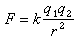"determines" the unit of electric charge (electric "fluid")if the coefficient of proportionality formally to assume to be equal to unit, k=1. Such definition is the phenomenological one because the Coulomb's law has been established without theoretical definition of the notion charge as the physical quantity, which may be either an amount of "fluid" or a quantity proportional to the last or a volumetric speed of "fluid" outflow, etc. It was the large-scale arbitrariness to assume that k =1, the irrational dimensionality of the charge demonstrates that. Following the above approach, we can formally assume also in the law of universal gravitationthat the gravitational constant is equal to unit, G =1. It has to be actually equivalent to the presentation of the law in the form of interaction of gravitational "fluids", their dimensionality coincides with dimensionality of the electric "fluid". The last indicates that the both "fluids" belong to the same irrational class of dimensionalities. The coefficient k in Coulomb's law (as well as the gravitational constant G in Newton's law of gravitation) expresses the definite parameters of the field and at k=1 the law, in fact, implicitly is rewrited in the following formthat determines the unit phenomenological quantity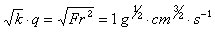and the charge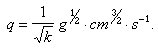. Consequently, the value of unit charge and its dimensionality remain unknown until the dimensionality class for k will not be determined. So, it is impossible to clear up the nature of electric field and charge without solving of the k-constant problem. Now, let us draw attention to the conventional "rationalization" of the Coulomb's law into SI units: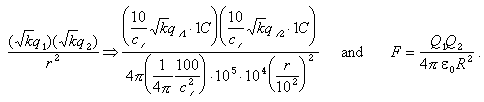Here, R is the distance in meters;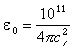is the constant of "rationalization" known as the electric constant,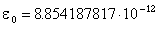(it is not equal to the absolute unit density of contents, designated earlier by the same symbol of GCS system, e0=1 g/s). This constant is dimensionless, but the nominative dimensionality dime0=F/m, that represents the pseudodimensionality because dim(F/m) =1, was attributed to it. Thus, we obtained one more constant, generated by errors of the past,. The spherical symmetry field of the charge must be reflected in Coulomb's law by the definite way. As has been convincingly shown by Smythe , this feature has to be presented in the law by the coefficient of 4p steradian. But as we see, the conventional "rationalization" is only visibility of it because the introduction of the quantity 4p has been accompanied with inserting of the inverse quantity 1/4p in the constant of "rationalization"e0. The error, connected with the coefficient of form, remains uneliminated until now as well as the presence of irrational charges with senseless dimensionalities. IV. A Philosophic-Physical Analysis of Coulomb's Law From viewpoint of general principles of philosophy and logic, Coulomb's law as an elementary law of physics has to be presented by the multiplicative expression composed of the constant and variable components. The constant component of the law has to be presented by the coefficients of form kf and contents kc . The variable component must be the functional one, its form was determined by Coulomb:, where q1, q2 are unknown dynamic measures of substance of field exchange. q1 = e0W1 and q2 = e0W2, whereW1 andW2 are kinematic measures and e0 = 1 g/cm3 is the absolute unit density of contents. As was mentioned above, the coefficient of form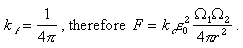. The quantity F is the dynamic one, consequently, the productmust be the dynamic quantity: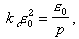, where p is assumed to be a zero-dimensional quantity. Accepting the last, we select by that the definite type of the kinematic quantity W and, thereby, the coefficient of contents takes the form:. Thus, we arrived at the philosophically justified form of Coulomb's law in which, at the field level, we will assume p =1 that has not a principal meaning because the such condition is stipulated by the scale reduction of measures W1 and W2 to the unit value p. As the final result, the Coulomb's law takes the following form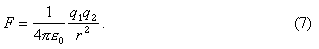From equation (7), we find that dimensionality of the "charge" q of the subatomic level of matter represents a mass speed of exchange at the field level since. As far as the mass power q and the Coulomb phenomenological charge qCGS are related by the equality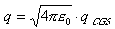then the elementary electron charge e, or the quantum of mass speed of exchange, will be equal to. If now to use the theoretical rational measure of the unit chargeand to introduce the metric coulomb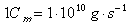then the electron charge can be presented by a triad of measures:which shows the advantage of the metric measures. Thus, the philosophic-logical solution of the problem of Coulomb's law structure on the basis of contents-form philosophy changes radically our conventional concepts about the microworld. In wave processes, the relation between the quantum of mass speed of exchange q and the quantum of mass of exchange m has the form q=wm, that is the analog of the relation between the amplitude speed of oscillations v and the displacement a: v=wa. If q=e and me are parameters of electron mass exchange then the characteristic exchange level of an "electrostatic" field is characterized by the fundamental frequency and fundamental wave radius: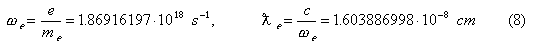The wave radiusis equal to one-half of mean value of interatomic distance in crystals. The theory of field material-ideal exchange by matter-space-time on the basis of the field of material-ideal numbers  gives for the quantum of mass of exchange, bearing in mind the Coulomb's law in the new form, the following expression:where r is the radius of a microparticle of the field,is the wave number; er = 1 at the field level. For an electron, k2r2<<1, hence its radius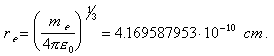The charge-power of exchange, corresponding to the mass of exchange (9), iswhere v is the velocity-strength of exchange at the surface of microparticle. Quanta of mass of exchange and of mass velocity of exchange are not the proper parameters of a microparticle, but they are parameters only material-ideal exchange-particle-field. In the theory of continuous media, masses of exchange are termed the associated masses. If to suppose that Bohr radius of a nucleon determines the boundary sphere of nucleon "atmosphere" then the associated mass of the nucleon will be equal to the following magnitude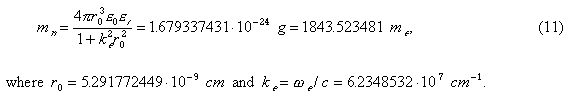The value mn obtained from (11) only bygreater than the mean value of mass attributed to a neutron, so that modern physics operates by exchange masses, which are the measures of interconnection of nucleons with a surrounding field of matter-space-time. In this field, nucleons are discrete objects with the radius of their own spherical spaces almost equal to the Bohr radius r0. Therefore, it is very difficult to image such structural atom units being in a "nucleus" of 10-13 cm size order if the last really exists. Everything this gives rise to state that atoms actually are neutron molecules. Their detailed structure has been considered in the work . Specifically the definite structure of nucleon molecules uniquely determines the definite form of corresponding crystals, i.e., it responds for the discrete space structure of any atomic formation. And when an atom is destroyed, it means that its space collapses. This results in the atomic energy release that has no respect to the atom nucleus, which does not exist, since in nodes of spherical nucleon molecules (atoms), as shown calculations , are no more than two neutrons. Now, it became clear where is the "Genetic Code" of structural variety in Nature.Bibliography  L.G. Kreidik, G.P. Shpenkov, Alternative Picture of the World, Volumes 1-3 (158, 164 and 186 pages, respectively), Bydgoszcz, 1996.  R. Danchev, A System Principle of Derivative Units (in Russian), Sofia, 1991.  W.R. Smythe, Static and Dynamic Electricity, New York - Toronto - London, 1950.  L.G. Kreidik, G.P. Shpenkov, The Material-Ideal Numerical Field, Proceeding of the Conference "CONTACT'95", Sofia, 1995, pp. 34-39.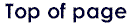Paideia logo design by Janet L. Olson. All Rights Reserved